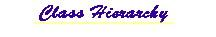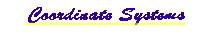## mil.navy.nps.rra Class Ray

```java.lang.Object
|
+--mil.navy.nps.rra.Ray
```

public class Ray
extends java.lang.Object

Ray implements the Recursive Ray Acoustics (rra) Algorithm derived by Professor Lawrence Ziomek to rapidly calculate a sound ray's 3D path through water, taking into account piecewise-local SSP, Surface and Bottom conditions.

Any wave front can be decomposed into a set of plane waves with the plane waves travelling in the direction of the normal to the wave front. The normal for each plane wave is defined by the normal to the wave front at the point where the front and the plane wave touch. The rra algorithm uses a difference-equation formulation of the ray theory solution to the linear acoustic wave equation. This provides a computationally rapid and theoretically general approach to simulate one ray on the surface of a wavefront as it propagates through the ocean. This makes rra an excellent choice to simulate underwater sonar under a very wide range of underwater environments.

Version:
1.0

Author:
LT Timothy M. Holliday USN

Contact: Don Brutzman (web.nps.navy.mil/~brutzman) brutzman@nps.navy.mil

Source Code:
~vrtp/mil/navy/nps/rra/Ray.java
http://devo.stl.nps.navy.mil/~brutzman/vrtp/mil/navy/nps/rra/Ray.javaHistory:
 1 Nov 97 Timothy M. Holliday New 17 Mar 98 Timothy M. Holliday Added HTML comment convention 12 Apr 98 Timothy M. Holliday Parameterless Constructors 14 Apr 98 Timothy M. Holliday Simplified VRML Routines 10 Oct 98 Don Brutzman Javadoc updates for jdk1.2b4 25 April 99 Don Brutzman Javadoc updates for jdk1.2.1

Reference:
Holliday, Timothy, Real-Time 3D Sonar Modeling and Visualization, Master's Thesis, Naval Postgraduate School, Monterey California, June 1998. Available at vrtp/rra/HollidayRRAthesis.pdf. and devo.stl.nps.navy.mil/~brutzman/vrtp/rra/HollidayRRAthesis.pdf. Slideset available in Powerpoint and Acrobat .pdf formats.

`ExampleRay`, `Beam`, `Lobe`

 Constructor Summary `Ray()`           Constructs a fixed size structure to trace a ray of acoustic energy through the ocean.

 Method Summary ` double` `getAbsorption()`           This method returns the current total relaxation absorbtion in dB. `static boolean` `getAppendLineFeed()`           This is a static method that returns the current line appendage. ` double` `getAzimuth()`           This method returns the azimuthal angle. ` Bottom` `getBottom()`           This method returns the handle to the bottom object. ` int` `getCount()`           This method returns the current number of points stored for the ray. ` double` `getDeltaTime()`           This method is returns the time step of the ray. ` int` `getDuration()`           This returns the ping duration. ` double` `getElevation()`           This method returns the elevation angle. ` Vec3d` `getNormal()`           This method returns the normal vector to the wavefront. ` Vec3d` `getPosition()`           This method gets the position of the ray. ` double` `getPositionX()`           This method returns the x-component of the position of the wavefront ` double` `getPositionY()`           This method returns the y-component of the position of the wavefront ` double` `getPositionZ()`           This method returns the z-component of the position of the wavefront ` double` `getReflectionPhase()`           This method returns the phase change of the wavefront ` SSP` `getSsp()`           This method returns the handle to the sound speed profile object. ` Surface` `getSurface()`           This method returns the handle to the surface object. ` double` `getTime()`           This method returns the current simulation time. ` double` `getTime(int index)`           This method returns a saved simulation time. ` Vec3d` `getTrailingPosition()`           This method returns the position of the trailing edge of the wavefront ` java.lang.String` ```normalizedTime(int N, double endtime)```           This method returns the requested normalized time as a string. ` java.lang.String` `position(int N)`           This method returns the requested position as a String. ` void` `Propagate(double timeStep)`           This method causes the ray to propagate one timestep into the future. ` void` `recordPoint()`           This method forces a recording of the current ray position. ` boolean` `reflected()`           This method returns whether or not the ray has been reflected in the most recent time step. ` void` `reset()`           This method resets all of the ray parameters after instantiation has occurred since reuse is more time efficient than garbage collection and reallocation. `static void` `setAppendLineFeed(boolean pAppendLineFeed)`           This is a static method used to indicate whether a line feed is desired at the end of every line. ` void` `setAzimuth(double phi)`           This method sets the azimuthal angle, which is the angle from the x-axis to the z-axis rotating about the y-axis. ` void` `setBottom(Bottom pBottom)`           This method sets the handle to the bottom object. ` void` `setDeltaTime(double deltaTime)`           This method is used to change the time step of the ray after instanciation. ` void` `setDuration(int duration)`           This method sets the ping duration. ` void` `setElevation(double beta)`           This method sets the elevation angle, which is the angle from the y-axis to the x-axis rotating about the z-axis. ` void` ```setPosition(double x, double y, double z)```           This method sets the position of the ray. ` void` `setSsp(SSP pSsp)`           This method sets the handle to the sound speed profile object. ` void` `setSurface(Surface pSurface)`           This method sets the handle to the surface object. ` double` `totalCurvature()`           This returns the total curvature of the ray path since the last recorded point. ` java.lang.String` `trailingPosition(int N)`           This method returns the requested trailing edge of the ray as a String.

 Methods inherited from class java.lang.Object ```, clone, equals, finalize, getClass, hashCode, notify, notifyAll, toString, wait, wait, wait```

 Constructor Detail

### Ray

`public Ray()`
Constructs a fixed size structure to trace a ray of acoustic energy through the ocean. Use accessor methods to set initial values of member variables.
 Method Detail

### reset

`public void reset()`
This method resets all of the ray parameters after instantiation has occurred since reuse is more time efficient than garbage collection and reallocation.

### getNormal

`public Vec3d getNormal()`
This method returns the normal vector to the wavefront.

### setAzimuth

`public void setAzimuth(double phi)`
This method sets the azimuthal angle, which is the angle from the x-axis to the z-axis rotating about the y-axis.

### getAzimuth

`public double getAzimuth()`
This method returns the azimuthal angle.

### setElevation

`public void setElevation(double beta)`
This method sets the elevation angle, which is the angle from the y-axis to the x-axis rotating about the z-axis.

### getElevation

`public double getElevation()`
This method returns the elevation angle.

### setPosition

```public void setPosition(double x,
double y,
double z)```
This method sets the position of the ray.

### getPosition

`public Vec3d getPosition()`
This method gets the position of the ray.

### setDeltaTime

`public void setDeltaTime(double deltaTime)`
This method is used to change the time step of the ray after instanciation.

### getDeltaTime

`public double getDeltaTime()`
This method is returns the time step of the ray.

### setDuration

`public void setDuration(int duration)`
This method sets the ping duration. Duration is currently 1 or 2. The integer refers to the number of deltaTime increments.

### getDuration

`public int getDuration()`
This returns the ping duration. Duration is currently 1 or 2. The integer refers to the number of deltaTime increments.

### setBottom

`public void setBottom(Bottom pBottom)`
This method sets the handle to the bottom object.

### getBottom

`public Bottom getBottom()`
This method returns the handle to the bottom object.

### setSurface

`public void setSurface(Surface pSurface)`
This method sets the handle to the surface object.

### getSurface

`public Surface getSurface()`
This method returns the handle to the surface object.

### setSsp

`public void setSsp(SSP pSsp)`
This method sets the handle to the sound speed profile object.

### getSsp

`public SSP getSsp()`
This method returns the handle to the sound speed profile object.

### getReflectionPhase

`public double getReflectionPhase()`
This method returns the phase change of the wavefront

### getPositionX

`public double getPositionX()`
This method returns the x-component of the position of the wavefront

### getPositionY

`public double getPositionY()`
This method returns the y-component of the position of the wavefront

### getPositionZ

`public double getPositionZ()`
This method returns the z-component of the position of the wavefront

### getTrailingPosition

`public Vec3d getTrailingPosition()`
This method returns the position of the trailing edge of the wavefront

### getTime

`public double getTime()`
This method returns the current simulation time.

### getTime

`public double getTime(int index)`
This method returns a saved simulation time.

### Propagate

`public void Propagate(double timeStep)`
This method causes the ray to propagate one timestep into the future.

### getCount

`public int getCount()`
This method returns the current number of points stored for the ray.

### getAbsorption

`public double getAbsorption()`
This method returns the current total relaxation absorbtion in dB.

### reflected

`public boolean reflected()`
This method returns whether or not the ray has been reflected in the most recent time step.

### recordPoint

`public void recordPoint()`
This method forces a recording of the current ray position. It also forces the total curvature of the ray since the last recorded point to 0.0.

### totalCurvature

`public double totalCurvature()`
This returns the total curvature of the ray path since the last recorded point. The curvature of a small segment of curve can be approximated by the curvature of a circle which is the change in angular position around the circle divided by the pathlength change. K = deltaTheta/deltaPathLength. Thus total curvature is the sum of the curvatures of the small segments along a ray trajectory.

### position

`public java.lang.String position(int N)`
This method returns the requested position as a String.

### trailingPosition

`public java.lang.String trailingPosition(int N)`
This method returns the requested trailing edge of the ray as a String.

### normalizedTime

```public java.lang.String normalizedTime(int N,
double endtime)```
This method returns the requested normalized time as a string.

### setAppendLineFeed

`public static void setAppendLineFeed(boolean pAppendLineFeed)`
This is a static method used to indicate whether a line feed is desired at the end of every line. 'true' indicates a linefeed is desired and 'false' indicates that a space is desired"

### getAppendLineFeed

`public static boolean getAppendLineFeed()`
This is a static method that returns the current line appendage.